# Colleges with the lowest SAT scores in Louisiana

Top 10 colleges in Louisiana with the lowest SAT scores
Looking for the colleges with the lowest SAT scores in Louisiana? Well you're in luck! We've compiled a national college database and have created a list of the top 10 universities with the lowest SAT scores in Louisiana below. If you are not a good test taker or worried about your test scores, this list is for you. These are the schools whose applicants had the lowest average SAT scores in Louisiana, which means that you can get into these colleges with a lower SAT score. We also include each college's ACT scores and acceptance rate so that you can see where you would have the easiest time getting in. Read on to find out more.

## University of Louisiana at Monroe SAT scores

The average SAT score for University of Louisiana at Monroe is 850.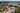The average SAT score of 850 breaks down into:

• SAT math: 440

The average ACT score for University of Louisiana at Monroe is 23 and their acceptance rate is 71.6%.

## Southern University at New Orleans SAT scores

The average SAT score for Southern University at New Orleans is 1010.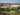The average SAT score of 1010 breaks down into:

• SAT math: 510

The average ACT score for Southern University at New Orleans is 19 and their acceptance rate is 37.2%.

## Xavier University of Louisiana SAT scores

The average SAT score for Xavier University of Louisiana is 1070.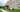The average SAT score of 1070 breaks down into:

• SAT math: 530

The average ACT score for Xavier University of Louisiana is 23 and their acceptance rate is 64.1%.

## Nicholls State University SAT scores

The average SAT score for Nicholls State University is 1120.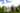The average SAT score of 1120 breaks down into:

• SAT math: 580

The average ACT score for Nicholls State University is 22 and their acceptance rate is 88.4%.

## Centenary College of Louisiana SAT scores

The average SAT score for Centenary College of Louisiana is 1140.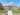The average SAT score of 1140 breaks down into:

• SAT math: 570

The average ACT score for Centenary College of Louisiana is 25 and their acceptance rate is 59.7%.

## University of Louisiana Lafayette SAT scores

The average SAT score for University of Louisiana Lafayette is 1140.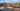The average SAT score of 1140 breaks down into:

• SAT math: 560

The average ACT score for University of Louisiana Lafayette is 23 and their acceptance rate is 55.3%.

## Southeastern Louisiana University SAT scores

The average SAT score for Southeastern Louisiana University is 1140.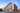The average SAT score of 1140 breaks down into:

• SAT math: 570

The average ACT score for Southeastern Louisiana University is 22 and their acceptance rate is 90.1%.

## University of New Orleans SAT scores

The average SAT score for University of New Orleans is 1140.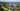The average SAT score of 1140 breaks down into:

• SAT math: 560

The average ACT score for University of New Orleans is 23 and their acceptance rate is 56.6%.

## Loyola University New Orleans SAT scores

The average SAT score for Loyola University New Orleans is 1157.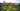The average SAT score of 1157 breaks down into:

• SAT math: 560

The average ACT score for Loyola University New Orleans is 25 and their acceptance rate is 94.3%.

## Louisiana Tech University SAT scores

The average SAT score for Louisiana Tech University is 1160.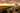The average SAT score of 1160 breaks down into:

• SAT math: 580